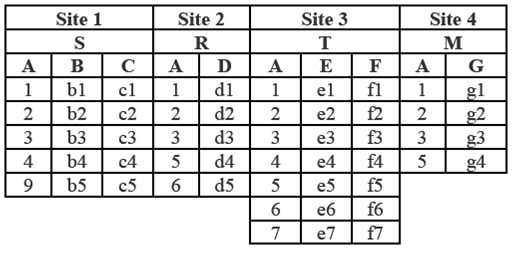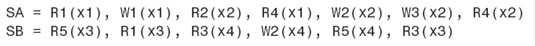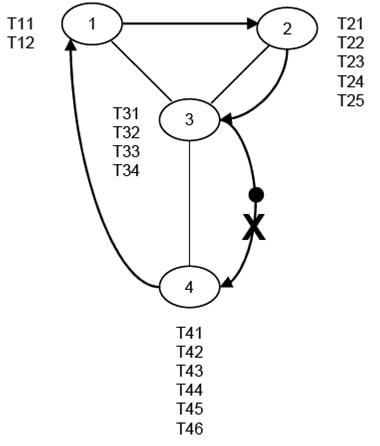# Research assignment question 1

1. Assume the cost of sending a message from any site to any other site is C units of time for SI (Semantic Integrity) validation. Figure out the cost of each one of the phases of the strategy.

Also assume that there are N transactions and, out of these, M will fail the validation. (20 points)

N * C which is the total cost of read-locking.

Phase 2: Control site performs the validation.

Cost for N validation = N*C

Phase 3: Invalid transactions are rejected—locks are released for these transactions.

There are N-M valid transaction, hence lock released = (N-M)*C

Phase 4: Control site calculates all the new values that need to be written.

None, as calculation is done by control site during these steps.

Phase 5: Control site write-locks information at all the sites it needs (no ACK for locking is required).

Cost = N*(RW )*C , without ACK

Phase 6: Control site writes where it needs to write for successful transactions.

(N-M)*(RW )*C

Phase 7: Control site unlocks at the sites that need to be unlocked.

Cost = M * (Ronly +Wonly + RW + SIS)*C, Cost for unlocking the sites that needs to be unlock

1. A four-site system has tables R, S, T, and M stored as depicted in Figure below. The user is at Site 1. Assume each row of any table that has more than one column can be sent in one message. You can also assume that a one-column table can be sent in one message. Also assume that the cost of sending each message is C units of time. There are no costs associated with sending commands. What is the cost of “(S JN R) JN (T JN M)” if “S JN R” and “T JN M” are done using semi-join and the final join is done normally? Make sure you show all steps and cost of communication for each step. (20 points)1. Assume transactions T1, T2, T3, T4, and T5 generate schedules SA and SB as shown below.

(A) Are the local SA and SB schedules serializable? If so, what are the equivalent local serial schedules? If not, why? (Show all partial commitment orders.)

(B) Are they globally serializable? If so, what is the equivalent global serial schedule? If not, why? (20 points)```{`
SA
Conflicts :                Dependency :
R1(X); W1(X)                T2 → T3
W1(X); R2(X)                T1 → T2
W2(X); R5(X)                T1 → T3
W3(X); R4(X)                T1 → T2
```

It is serializable as there is no cycle.

```    SB

Conflicts :                Dependency :
R5(X); R1(X)                T2
R3(X); W2(X)                T2 → T3
R4(X); W2(X)                T3 → T2
`}```

It is not serializable as there is cycle.

1. This problem is a continuation from Problem 7.3 (page 294). Please make sure to read Figure 7.8 on page 294 for Cycle 1 before doing this problem. Assume that when a site receives the token it can ticket a maximum of three transactions, and it will ticket three transactions when there are at least three transactions waiting at the site. As depicted in Figure below, during the second Cycle, the communication line between Site 3 and 4, when the token is on it, goes down. For this cycle, answer the following questions: (20 points)1. What is the ticket number when the token leaves Site 1?
2. What is the ticket number when the token comes back to Site 1?
3. How many transactions, and in what order, are serialized at the completion of cycle 1 and cycle 2?
1. For the specific research area that you surveyed for your research project, what are the major challenge(s) that will influence the deployment of distributed database? How would you address those challenge(s)? (20 points)